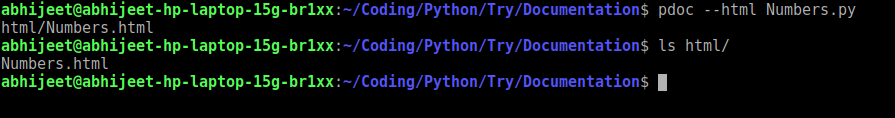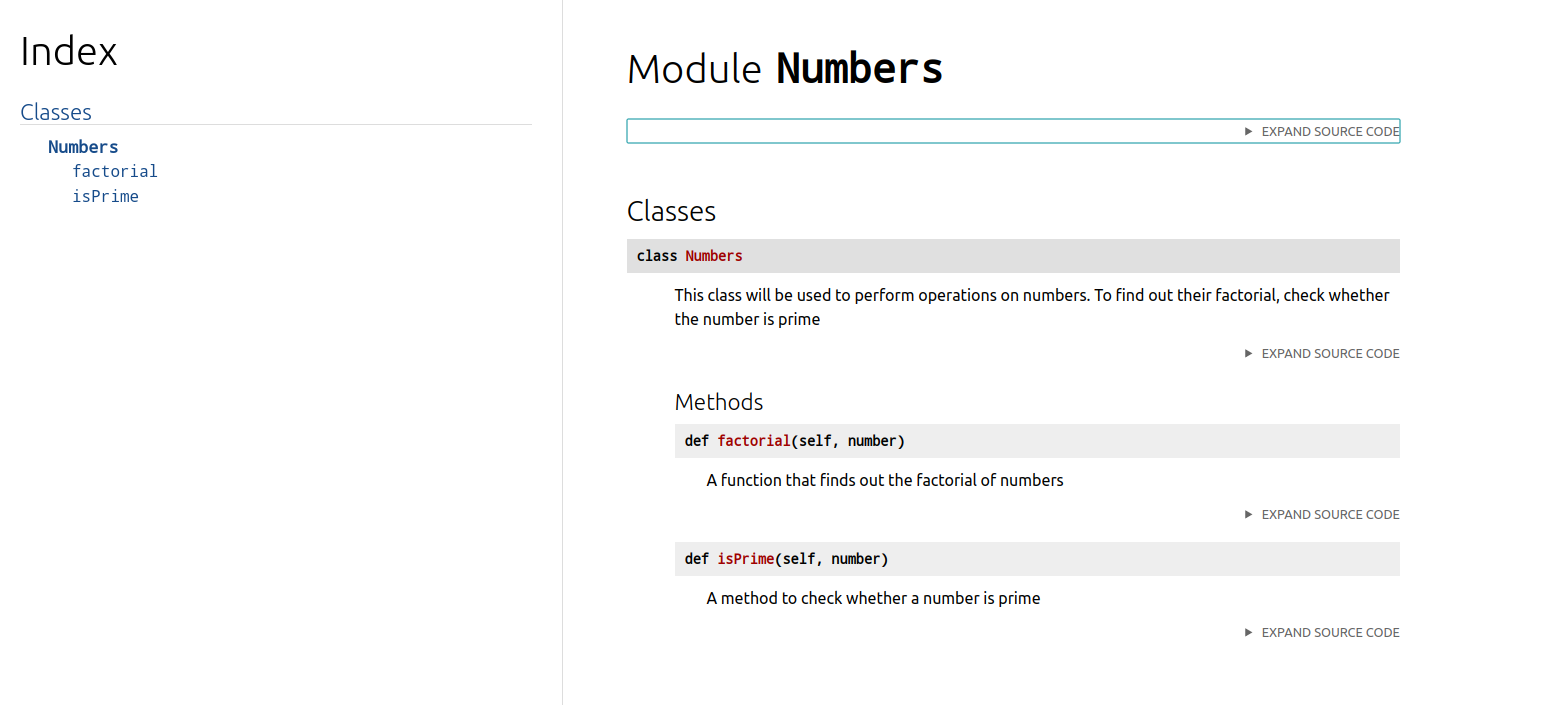# How to generate a documentation using Python?

• Last Updated : 01 Oct, 2020

Documentation improves the readability of the code. There are many tools that help us to create documentations. One such tool is pdoc to write documentation for python projects.

Installation: Run the following pip command on the terminal.

```pip3 install pdoc3
```

Now navigate (through command line) to the folder where our Python program is kept. Let’s say the name of our program is Numbers.py. It is a simple class that has 2 functions, one to find the factorial of the number and another to check whether the number is a prime number or not.

## Python3

 `class` `Numbers :``  ` `        ``"""``        ``This class will be used to perform operations on numbers.``        ``To find out their factorial, check whether the number is prime``        ``"""``  ` `        ``def` `isPrime(``self``, number) :``  ` `                ``'''``                ``A method to check whether a number is prime``                ``'''``  ` `                ``i ``=` `2``                ``while``(i <``=` `number ``/` `2``) :``                        ``if` `number ``%` `i ``=``=` `0``:``                                ``return` `False``                        ``i ``+``=` `1``                ``return` `True``         ` `        ``def` `factorial(``self``, number) :``             ` `                ``"""``                ``A function that finds out the factorial of numbers``                ``"""``                ``result ``=` `1``                ``while``(number >``=` `2``) :``                        ``result ``*``=` `number``                        ``number ``-``=` `1``                ``return` `result`

Adding docstrings in classes and methods provide a convenient way of associating documentation with Python modules, functions, classes, and methods. Docstrings are an important part of the documentation.

After navigating to the folder(through command line) where our code (Numbers.py) is kept. We have to type this command in the terminal:

``` pdoc --html Numbers.py
```Directory structure

After firing the –html command shown above, an HTML folder will be created in the directory where Numbers.py is kept. Inside that HTML folder, a file called Numbers.html will be created. Navigate to HTML folder and open Numbers.html.Numbers.html

Numbers.html should be looking like this. We just created proper documentation of our class Numbers. In the left column(Index) of Number.html, classes and it’s methods are seen. Remember the docstrings which we wrote, they are available below their respective classes and methods.

My Personal Notes arrow_drop_up